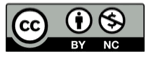# Python 用递归生成器计算笛卡尔积

2014-06-26## 笛卡尔积的含义

$A$$B$$C$
$a_1$$b_1$$c_1$
$a_2$$b_2$$c_2$
$a_3$

123456789101112
$a_1$$a_1$$a_1$$a_1$$a_2$$a_2$$a_2$$a_2$$a_3$$a_3$$a_3$$a_3 b_1$$b_1$$b_2$$b_2$$b_1$$b_1$$b_2$$b_2$$b_1$$b_1$$b_2$$b_2$
$c_1$$c_2$$c_1$$c_2$$c_1$$c_2$$c_1$$c_2$$c_1$$c_2$$c_1$$c_2$

## 内置函数 product( )

Python内置的 itertools.product()函数 可以得到N个向量的笛卡尔积，例如：

from itertools import product
list(product([1,2,3],,[5,6,7]))
# [(1, 4, 5), (1, 4, 6), (1, 4, 7), (2, 4, 5), (2, 4, 6),\
# (2, 4, 7), (3, 4, 5), (3, 4, 6), (3, 4, 7)]


product()函数 返回一个生成器，它的等效代码为：

def product(*args, **kwds):
# product('ABCD', 'xy') --> Ax Ay Bx By Cx Cy Dx Dy
# product(range(2), repeat=3) --> 000 001 010 011 100 101 110 111
pools = map(tuple, args) * kwds.get('repeat', 1)
result = [[]]
for pool in pools:
result = [x+[y] for x in result for y in pool]
for prod in result:
yield tuple(prod)


## 生成器思路

def 剥(任意一颗洋葱)


def 剥(洋葱):
如果 洋葱剥没了:
什么也给不了
否则:
剥去最外层
剥(剩下的洋葱)


def 笛卡尔积(序列):
如果 序列 为 空:
给出（yield）空序列
否则:
拆开第一个子序列，对于其中每个元素
把这个元素加在 笛卡尔积(剩下的序列) 的每一个结果 前面
给出（yield） 这个组合


## 用python语言实现递归生成器

def combi(seq):
if not seq:
yield []
else:
for element in seq:
for rest in combi(seq[1:]):
yield [element] + rest


n=[[1,2,3],,[5,6,7]]
print list(combi(n))


## 应用举例

[[1,2,3],,[5,6,7]]


拆出第一个序列，得到1,2,3

对于4                      （暂存结果[1, 4]）
拆出第3个序列，得到5,6,7
对于5                       （暂存结果[1, 4, 5]）
拆出第4个序列（空），得到（空）
把5加到（空）前面，返回结果    （返回暂存结果[1, 4, 5]）
对于6                       （暂存结果[1, 4, 6]）
拆出第4个序列（空），得到（空）
把6加到（空）前面，返回结果    （返回暂存结果[1, 4, 6]）
对于7                       （暂存结果[1, 4, 7]）
拆出第4个序列（空），得到（空）
把7加到（空）前面，返回结果    （返回暂存结果[1, 4, 7]）


[1, 4, 5], [1, 4, 6], [1, 4, 7]


## 精彩评论